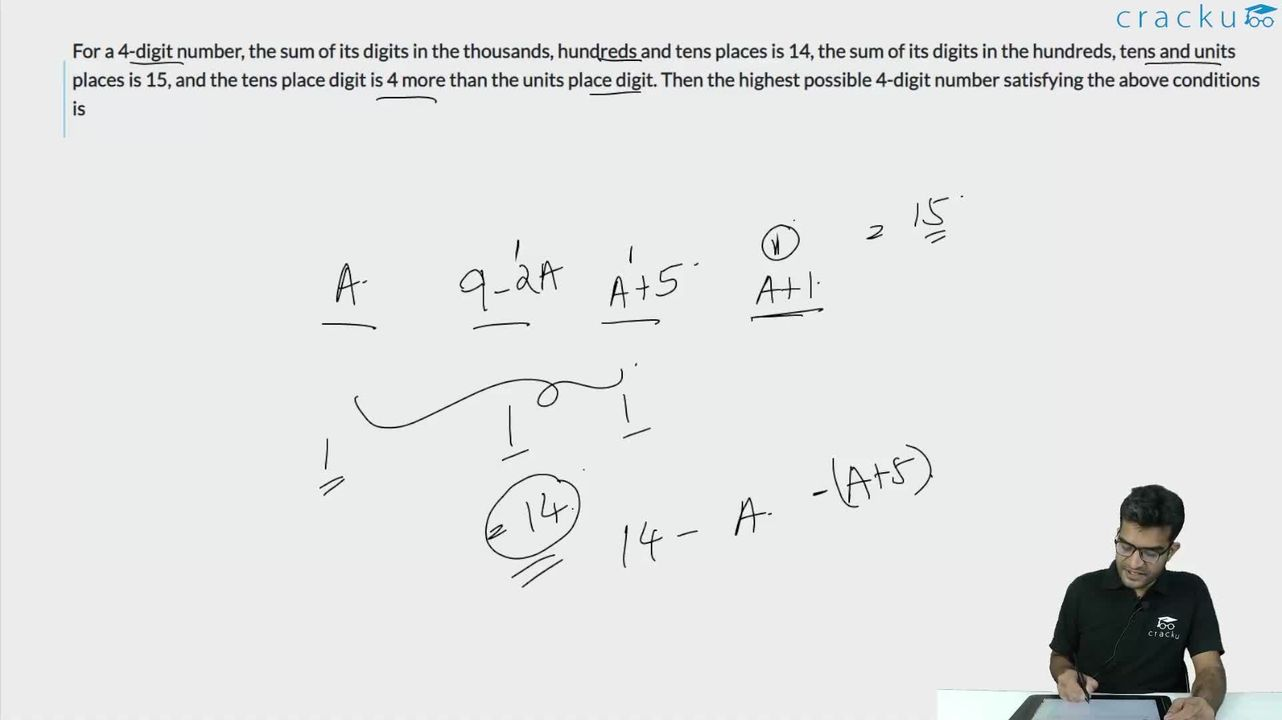Question 47

# For a 4-digit number, the sum of its digits in the thousands, hundreds and tens places is 14, the sum of its digits in the hundreds, tens and units places is 15, and the tens place digit is 4 more than the units place digit. Then the highest possible 4-digit number satisfying the above conditions is

Solution

Given the 4 digit number :

Considering the number in thousands digit is a number in the hundredth digit is b, number in tens digit is c, number in the units digit is d.

Let the number be abcd.

Given that a+b+c = 14. (1)

b+c+d = 15. (2)

c = d+4. (3).

In order to find the maximum number which satisfies the condition, we need to have abcd such that a is maximum which is the digit in thousands place in order to maximize the value of the number. b, c, and d are less than 9 each as they are single-digit numbers.

Substituting (3) in (2) we have b+d+4+d = 15, b+2*d = 11.  (4)

Subtracting (2) and (1) : (2) - (1) = d = a+1.   (5)

Since c cannot be greater than 9 considering c to be the maximum value 9 the value of d is 5.

If d = 5, using d = a+1, a = 4.

Hence the maximum value of a = 4 when c = 9, d = 5.

Substituting b+2*d = 11. b = 1.

The highest four-digit number satisfying the condition is 4195

### View Video Solution